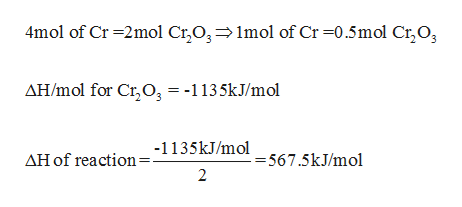Calculate the change in the enthalpy when 52.0 g of solid chrominium at 25*C and 1 atm pressure is oxidized. (Delta Hf for Cr2O3(s) is -1135 Kj/Mol.)4Cr(S) +3 O2(g) = 2Cr2O3 (s). A. -1135B. -284 KjC. -568 KjD. 1135 KjE. 568 Kj

Question

Calculate the change in the enthalpy when 52.0 g of solid chrominium at 25*C and 1 atm pressure is oxidized. (Delta Hf for Cr2O3(s) is -1135 Kj/Mol.)

4Cr(S) +3 O2(g) = 2Cr2O3 (s).

A. -1135

B. -284 Kj

C. -568 Kj

D. 1135 Kj

E. 568 Kj

Step 1

Molar mass of chromium is 52g/mol, which means 1mol of Cr = 52g of Cr.  According to stoichiom...help_outlineImage Transcriptionclose4mol of Cr 2mol Cr,03 1mol of Cr -0.5mol Cr,O AH/mol for Cr O3 = -1135kJ/mol AH of reaction:1135kJ/mol _567.5kJ/mol = 2 fullscreen

Want to see the full answer?

See Solution

Want to see this answer and more?

Our solutions are written by experts, many with advanced degrees, and available 24/7

See Solution
Tagged in

General Chemistry